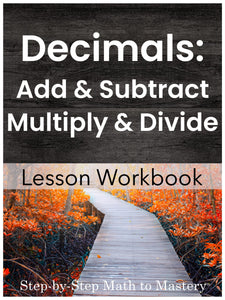# Decimals: Add, Subtract, Multiply & Divide

• \$12.00
Unit price per
Shipping calculated at checkout.

## Adding and Subtracting Decimals, Multiplying and Dividing Decimals, Decimal Word Problems

### 23 Step-by-Step Math Lessons | Special Education Math Intervention | Fifth Grade Math Level

This Adding and Subtracting Decimals and Multiplying and Dividing Decimals lesson workbook includes an example IEP goal, 23 step-by-step math lessons, reviews and assessments. This evidence-based math intervention is tied to fifth grade standards and is great for special education math goals and tier 2 small group math interventions (RTI).

These sequential lessons are easy to teach, with enough material for 5+ weeks of instruction!

This lesson workbook covers decimal addition, subtraction, multiplication, & division. Decimal numbers go to the tenths and hundredths places.  Scripted model problems make it easy to teach clear, sequential lessons. Skills are introduced incrementally.  Before beginning these lessons, I recommend students complete  “Decimal Basics: Read, Write, Compare, and Round”.

23 Lessons
3 Reviews3 Tests

183 Pages

5+ Weeks of Instruction

## Students Will Be Able To:

• Add and subtract numbers to the same decimal place
• Write equivalent decimals to add and subtract
• Rewrite problems vertically by lining up the decimal points
• Add a whole number and mixed decimal
• Subtract a decimal from a whole number
• Solve word problems involving adding and subtracting decimals

Multiply and Divide Decimals

• Multiply decimals and place the decimal point in the product correctly
• Multiply decimals by 10 and 100
• Divide a decimal by a whole number
• Divide a whole number by a whole number; show the remainder as a decimal.
• Divide and round off answers to the nearest tenth or hundredth.
• Divide when the divisor is a decimal.
• Solve word problems involving multiplying and dividing decimal numbers.

## Lessons Are Tied to These Standards:

Add, subtract, multiply, and divide decimals to hundredths, using concrete models or drawings and strategies based on place value, properties of operations, and/or the relationship between addition and subtraction; relate the strategy to a written method and explain the reasoning used.

Explain patterns in the number of zeros of the product when multiplying a number by powers of 10, and explain patterns in the placement of the decimal point when a decimal is multiplied or divided by a power of 10.

Fluently add, subtract, multiply, and divide multi-digit decimals using the standard algorithm for each operation.

### Not sure where to start?

Watch a video overview of Step-by-Step Math to Mastery or read the transcript here.

### Step-by-Step Math to Mastery™ Resources:

Make math easier to understand.

• Help students over math hurdles with clear, sequential, scaffolded lessons.
• Prevent overwhelm. Build student confidence.

Make math easier to teach.

• Save hours of planning and piecing together materials.
• Paraeducators can deliver quality instruction independently. Open & teach.

### Reasons You'll Love Step-by-Step Math to Mastery™ Resources

• Boosts student confidence and progress
• Time-saving: print and teach
• Easy prep: only black ink is needed
• Easy to teach, paraeducator-friendly
• Example IEP goals and shorter term objectives
• Consistent & predictable format
• Lots of practice repetitions
• Scaffolded with structured workspaces
• Fewer problems on a page, white space, minimal visual clutter
• Tied to standards
• Explicit/Direct instruction
• Systematic & sequential
• Mastery approach--teaching one topic at a time, one strategy at a time
• Lessons have "I Can" statements, model problems, guided practice, & independent practice
• Each workbook can be used individually as a stand-alone intervention for that skill or they can be used together, taking students from the basics of number sense and addition in first grade all the way to dividing fractions and decimals in fifth grade.
• Can also be used with older students (middle & high school) to help fill the gaps in their learning.